# Pentacontagon

Regular pentacontagon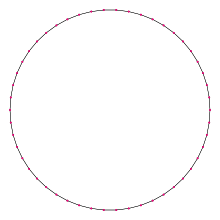A regular pentacontagon
Type Regular polygon
Edges and vertices 50
Schläfli symbol {50}, t{25}
Coxeter diagramSymmetry group Dihedral (D50), order 2×50
Internal angle (degrees) 172.8°
Dual polygon Self
Properties Convex, cyclic, equilateral, isogonal, isotoxal

In geometry, a pentacontagon or pentecontagon or 50-gon is a fifty-sided polygon. The sum of any pentacontagon's interior angles is 8640 degrees.

A regular pentacontagon is represented by Schläfli symbol {50} and can be constructed as a quasiregular truncated icosipentagon, t{25}, which alternates two types of edges.

## Regular pentacontagon properties

One interior angle in a regular pentacontagon is 17245°, meaning that one exterior angle would be 715°.

The area of a regular pentacontagon is (with t = edge length)The circumradius of a regular pentacontagon isSince 50 = 2 × 52, a regular pentacontagon is not constructible using a compass and straightedge, and is not constructible even if the use of an angle trisector is allowed.

## Symmetry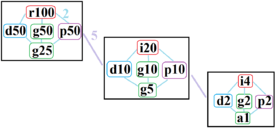The symmetries of a regular pentacontagon. Light blue lines show subgroups of index 2. The 3 boxed subgraphs are positionally related by index 5 subgroups.

The regular pentacontagon has Dih50 dihedral symmetry, order 100, represented by 50 lines of reflection. Dih50 has 5 dihedral subgroups: Dih25, (Dih10, Dih5), and (Dih2, Dih1). It also has 6 more cyclic symmetries as subgroups: (Z50, Z25), (Z10, Z5), and (Z2, Z1), with Zn representing π/n radian rotational symmetry.

John Conway labels these lower symmetries with a letter and order of the symmetry follows the letter. He gives d (diagonal) with mirror lines through vertices, p with mirror lines through edges (perpendicular), i with mirror lines through both vertices and edges, and g for rotational symmetry. a1 labels no symmetry.

These lower symmetries allows degrees of freedom in defining irregular pentacontagons. Only the g50 subgroup has no degrees of freedom but can seen as directed edges.

## Pentacontagram

A pentacontagram is a 50-sided star polygon. There are 9 regular forms given by Schläfli symbols {50/3}, {50/7}, {50/9}, {50/11}, {50/13}, {50/17}, {50/19}, {50/21}, and {50/23}, as well as 16 compound star figures with the same vertex configuration.

 Picture Interior angle Picture Interior angle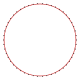{ 50⁄3}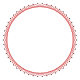{ 50⁄7}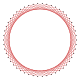{ 50⁄9}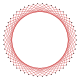{ 50⁄11}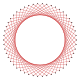50⁄13 158.4° 129.6° 115.2° 100.8° 86.4°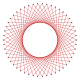{ 50⁄17 }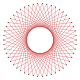{ 50⁄19 }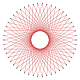{ 50⁄21 }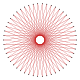{ 50⁄23 } 57.6° 43.2° 28.8° 14.4°
1. Gorini, Catherine A. (2009), The Facts on File Geometry Handbook, Infobase Publishing, p. 120, ISBN 9781438109572.
2. Constructible Polygon
3. http://www.math.iastate.edu/thesisarchive/MSM/EekhoffMSMSS07.pdf
4. The Symmetries of Things, Chapter 20